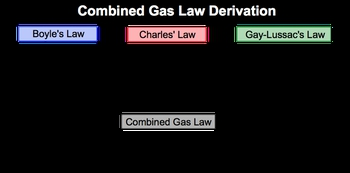## GOB Chemistry

Learn the toughest concepts covered in your GOB - General, Organic, and Biological Chemistry class with step-by-step video tutorials and practice problems.

Gases, Liquids and Solids

# Chemistry Gas Laws: Combined Gas Law

The Combined Gas Law is created from “combining” Boyle’s Law, Charles’ Law, and Gay–Lussac’s Law.

Combined Gas Law
1
concept

## Chemistry Gas Laws: Combined Gas Law1m
Play a video:
now if we have the combined gas law. The combined gas law was created from combining Boyle's law, Charles Law and Gay Loose Ex Law. We're gonna say it highlights the relationship between the variables off pressure, volume and temperature. Remember, Boyle's Law says that pressure is inversely proportional to volume Charles losses. That volume is directly proportional. It's a temperature and gay loose X losses. That pressure is directly proportional to temperature, and we take a look down here. We could figure out how the combined gas law was derived. So it comes from combining Boyle's long Charles law and gay loose ax law. If we look, we have pressure and volume as numerator. So volume here numerator pressure here. Numerator. So they share those variables in common with one another so that B p v as enumerators, Charles law and gay loose sacks law have as their denominators temperature. So that comes down here. So here we say that the combined gas law is PV over T equals some constant. So okay, here would be a constant. This year would represent our combined gas law. And if we're dealing with two sets of pressures, volumes and temperature, then it can go further and say p one v one over t one equals p two V two over t to So this is how the combined gas law can be derived from these earlier chemistry gas laws.2
example

## Chemistry Gas Laws: Combined Gas Law Example 13m
Play a video: